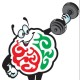# 2011 April LET Review: Yet Another 10 Math Questions To Solve

Good afternoon, guys! Here is another set of Math word problems to solve. The following problems are similar to the ones posted before.

1. A department store advertises a 25%-off sale. A pair of jeans on sale is marked P28.50. What was the former price?

2. The value of a fraction is 5/8. If the sum of the numerator and denominator is 65, what is the numerator?

3. The sum of the digits of a two-digit number is 12. If 6 is subtracted from the number, the result is ½ the number formed by reversing the digits of the original number. What is the original number?

4. A motorboat took 5 hours to make a downstream trip with a 15-mph current. The return trip against the same current took 8 hours. How fast is the boat in still water?

5. In a housing project Ronnie can paint a house in 2 months. If Damien helps him, they can finish painting 5 houses in 6 months. If he works alone, how long will Damien finish painting a house?

6. Anna has twice as many dimes as nickels, and 12 more quarters than the number of dimes and nickels together. If she had \$5.00 in all, how many nickels, dimes, and quarters did she have?

7. In 7 years, Dianne’s age will exceed JP’s age by 14 years. Last year, the sum of their ages was 20. How old is Dianne?

8. Pedro, Juan, and Pepe mixed 10 liters of a 6% alcohol solution with a 24% alcohol solution in order to get 15% solution. How many liters of the 24% solution are needed?

9. There are two consecutive even integers, where three times the first number is 20 more than twice of the second number. What are the integers?

10. There are 35 cows and chickens in a farm. If the caretaker counted 100 animal feet, how many chickens are there?

Done solving? Here are the answers!

1. P38

2. 25

3. 48

4. 65 mph

5. 3

6. 3 nickels, 6 dimes, 20 quarters

7. 18

8. 10 liters

9. 24 and 26

10. 20

If you have any queries, just use the comment box below 🙂